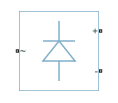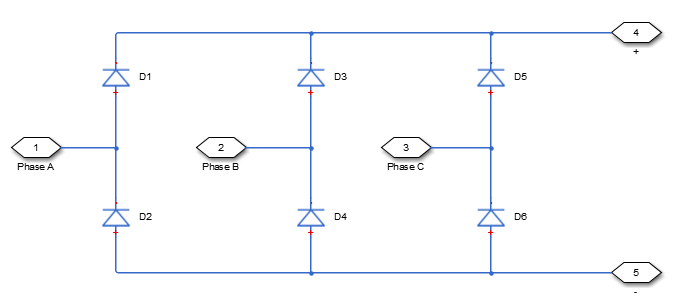# Average-Value Rectifier (Three-Phase)

Average-value three-phase AC voltage to DC voltage converter with fixed power loss

• Library:
• Simscape / Electrical / Semiconductors & Converters / Converters

•## Description

The Average-Value Rectifier (Three-Phase) block models an average-value, full-wave, six-pulse rectifier. It converts instantaneous three-phase AC voltages to DC voltage and DC power demand to three-phase AC power demand. The corresponding AC power demand is equal to the sum of the fixed power loss and the DC power demand.

You can use the Average-Value Rectifier (Three-Phase) block only as a six-pulse rectifier. You cannot combine two Average-Value Rectifier blocks to represent a twelve-pulse rectifier.

The figure shows the equivalent circuit for the rectifier as a full-wave, six-pulse rectifier. The Average-Value Rectifier (Three-Phase) block does not yield the harmonics that are typically associated with the detailed representation, however, because it performs an average-value power conversion.This block can work in both time and frequency-and-time simulation modes. If you set the AC frequency parameter to `Variable`, this block works only in time simulation mode. If you select `Constant`, this block works in both time and frequency-time simulation modes. For more information, see Frequency and Time Simulation Mode.

### Electrical Defining Equations

The voltages are defined by:

`${v}_{ref}=\frac{{v}_{a}+{v}_{b}+{v}_{c}}{3},$`

`${V}_{RMS}=\sqrt{\frac{{\left({v}_{a}-{v}_{b}\right)}^{2}+{\left({v}_{b}-{v}_{c}\right)}^{2}+{\left({v}_{c}-{v}_{a}\right)}^{2}}{3}},$`

`${v}_{DC}=3\frac{\sqrt{2}}{\pi }{V}_{RMS},$`

`${v}_{p}={v}_{ref}+\frac{{v}_{DC}}{2},$`

and

`${v}_{n}={v}_{ref}-\frac{{v}_{DC}}{2},$`

where:

• va, vb, vc are the respective AC phase voltages.

• vref is the DC offset on the AC side. In a balanced AC power system with no DC bias, vDC is `0` V.

• VRMS is the RMS AC line-line voltage.

• vDC is the voltage difference between the positive and negative terminals of the rectifier.

• $3\sqrt{2}/\pi$ is the vDC /VRMS ratio for a full-wave, six-pulse rectifier.

• vp, vn are the voltages at the positive and negative terminals of the rectifier.

The resistance, power, and currents are defined by

`${R}_{fixed}=\frac{{V}_{Rated}^{2}}{{P}_{fixed}},$`

`${P}_{DC}=-{v}_{p}{i}_{p}-{v}_{n}{i}_{n},$`

`${R}_{AC}=\frac{{V}_{RMS}^{2}}{{P}_{DC}+\frac{{V}_{RMS}^{2}}{{R}_{fixed}}},$`

and

`$\left[\begin{array}{ccc}{i}_{a}& {i}_{b}& {i}_{c}\end{array}\right]=\frac{\left[\begin{array}{ccc}{v}_{a}& {v}_{b}& {v}_{c}\end{array}\right]-{v}_{ref}}{{R}_{AC}},$`

where:

• VRated is the rated AC voltage that you specify on the block mask.

• Pfixed is the fixed power loss that you specify on the block mask.

• Rfixed is the fixed per-phase series resistance in an equivalent wye-connected load.

• ip, in are the currents flowing into the positive and negative terminals of the rectifier.

• PDC is the power output on the DC side. PDC has a minimum limit of `0` W.

• RAC is the per-phase series resistance in an equivalent wye-connected load.

• ia, ib, ic are the respective AC phase currents flowing into the rectifier.

## Ports

### Conserving

expand all

#### Dependencies

To enable this port, set Electrical connection to ```Composite three-phase ports```.

Electrical conserving port associated with a-phase.

#### Dependencies

To enable this port, set Electrical connection to ```Expanded three-phase ports```.

Electrical conserving port associated with b-phase.

#### Dependencies

To enable this port, set Electrical connection to ```Expanded three-phase ports```.

Electrical conserving port associated with c-phase.

#### Dependencies

To enable this port, set Electrical connection to ```Expanded three-phase ports```.

Electrical conserving port associated with the positive terminal.

Electrical conserving port associated with the negative terminal.

## Parameters

expand all

Whether to have composite or expanded three-phase ports.

Whether to have a variable or constant AC frequency:

• `Variable` — If the frequency at the AC side varies, this option only works in time simulation mode.

• `Constant` — If the frequency at the AC side is constant, this option works in both time and frequency-and-time simulation modes.

Rated voltage of the AC system.

Rated electrical frequency. The value of this parameter must be equal to the value of the rated electrical frequencies of other sinusoidal sources in your model, if present.

#### Dependencies

To enable this parameter, set AC frequency to `Constant`.

Minimum power drawn on the AC side at rated AC voltage. When the instantaneous AC voltage is equal to the value you specify for the Rated AC voltage, the AC power demand equals the value you specify for the Fixed power loss plus DC power demand.

## Version History

Introduced in R2014b

expand all